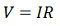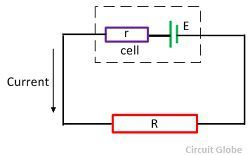# Difference Between EMF and Voltage

One of the major difference between the EMF and voltage is that EMF is the energy supplied to the charge, whereas the voltage is the energy requires to move the unit charge from one point to another. The other differences between them are explained below in the comparison chart.

## Comparison Chart

Basis for ComparisonEMFVoltage
DefinitionThe amount of energy supply by the source to each coulomb of charge.Energy use by unit charge to move from one point to another
FormulaSymbolε V
MeasureMeasure between the end point of the source, when no current flows through it.Measure between any two points.
SourceDynamo, electrochemical cell, transformer, solar cell, photodiodes etc.Electric and magnetic field

### Definition of Voltage

Voltage is defined as the energy requires to moves the unit charge from one point to another. It is measured in volts and represented by the symbol V. The voltage is caused by the electric and the magnetic field.

The voltage is developed between the ends (i.e. the cathode and anode) of the source. The potential of the positive endpoint of the source is higher as compared to the negative points. When the voltage develops across the passive element, then it is called the voltage drops. The sum of the voltage drops in a circuit is equal to the EMF according to Kirchoff’s law.

### Definition of EMF

The energy supply from the source to each coulomb of charge is known as the EMF. In other words, it is the energy supply by some active source such as the battery to the unit coulomb charge. The EMF stands for the electromotive force. It is measured in volts and represented by the symbol ε.The electromotive force of the above circuit is represented by the formulaWhere, r – internal resistance of the circuit.
R – External resistane of the circuit.
E – electromotive force.
I – current

## Key Differences Between EMF and Voltage

1. The EMF is the measure of energy supply to each coulomb of charge, whereas the voltage is the energy use by one coulomb of charge to move from one point to another.
2. The EMF is represented by the ε whereas the symbolic representation of the voltage is V.
3. The EMF is measured between the end point of the source, when no current flow through it, whereas, the voltage is measured between any two points of the closed circuit.
4. The EMF is generated by the electrochemical cell, dynamo, photodiodes, etc., whereas the voltage is caused by the electric and magnetic field.

Volt is the SI unit of both the EMF and the voltage.

### 7 thoughts on “Difference Between EMF and Voltage”

1. Your content is extremely fruitful for students.
Thanks for this anticipation
Yours obediently,
Jamil Akhter.